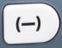# Scientific NotationBasics
Scientific notation is used to represent large or small numbers as a power of ten.

Scientific notation is constructed in a series of steps:
(1)  Place the decimal point to the right of the first non-zero digit.
(2)  Count the number of places the decimal point has moved.
(3)  Use the number of places moved as a power of ten.
[If the original number is 10 or more, the power of ten will be a positive number.]
[If the original number is less than 1, the power of ten will be a negative number.]

When dividing a number in scientific notation by another number in scientific notation, parentheses are often needed to protect the order of operations.

The word fit is often associated with a division question.is the scientific notation command.

Question

What is 7200000 expressed in scientific notation?

7.2 × 106

(1)  Place the decimal point to the right of the first non-zero digit.
7200000 —> 7.200000

(2)  Count the number of places the decimal point has moved.
7.200000 <— 7200000.
The decimal point has moved 6 places from right to left.
[Remember from your knowledge of decimals that there is an invisible decimal point to the right of a whole number, such that 7200000. = 7200000]

(3)  Use the number of places moved as a power of ten.
Because the original number is 10 or more, the power of ten will be a positive number.
7200000 —> 7.200000 = 7.2 × 106

Question
What is 0.0000072 expressed in scientific notation?

7.2 × 10-6

(1)  Place the decimal point to the right of the first non-zero digit.
.0000072 —> 000007.2
[Remember from your knowledge of decimals that 0.0000072 contains a placeholder zero, such that 0.0000072 = .0000072]

(2)  Count the number of places the decimal point has moved.
.0000072 —> 000007.2
The decimal point has moved 6 places from left to right.

(3)  Use the number of places moved as a power of ten.
Because the original number is less than 1, the power of ten will be a negative number.
.0000072 —> 000007.2 = 7.2 × 10-6

Question

According to the calculator, what is the value of 9.5 × 108 ÷ 4.75 × 10-7?
According to the calculator, what is the value of (9.5 × 108) ÷ (4.75 × 10-7)?
Observe how parentheses change the results of these calculations.

9.5 × 108 ÷ 4.75 × 10-7 = 20
(9.5 × 108) ÷ (4.75 × 10-7) = 2.0 × 1015

The second calculation illustrates the way parentheses protect the order of operations when scientific notation involves division.

Calculator Click What You See Commentblinker clears screen
9.58÷
4.7579.5*108÷4.75*10-7 Division20 Answerblinker clears screen9.58÷4.757(9.5*108)÷(4.75*10-7) Division

###### Parentheses protect order of operations.2×1015 Answer
###### Scientific Notation

Question
A transistor is 6.0 × 10-8 mm wide.  If  a microchip is 12 mm wide, how many transistors can be fitted into the microchip side by side?  Express your answer in scientific notation.

2.0 × 108

To get the correct answer, you must use parentheses to protect the order of operations.
(12) ÷ (6.0*10-8) = 200000000 = 2.0 × 108

Calculator Click What You See Commentblinker clears screen12÷6.08(12)÷(6.0*10-8) Division

###### Parentheses protect order of operations.200000000 Answer
(before scientific notation)
###### Scientific Notation

Practice – Questions
1.  What is 10000000 expressed in scientific notation?

2.  What is 0.0000001 expressed in scientific notation?

3.  What is 2945000000 expressed in scientific notation?

4.  What is 0.000000002945 expressed in scientific notation?

5.  What is 1.58 × 102 ÷ 3.2 × 10-5?  Express your answer in decimal form.

6.  With reference to Question 5, express your answer in scientific notation.

7.  What is (1.58 × 102) ÷ (3.2 × 10-5)?  Express your answer in decimal form.

8.  With reference to Question 7, express your answer in scientific notation.

9.  A transistor is 3.2 × 10-8 mm wide.  If  a microchip is 25 mm wide, how many transistors can be fitted into the microchip side by side?  Express your answer in scientific notation.

10.  A gamma ray is 1.0 × 10-12 mm wide.  If  visible light is 7.0 × 10-7 mm wide, how many gamma rays can be fitted into the same space occupied by visible light?  Express your answer in scientific notation.

1.  1.0 × 107

2.  1.0 × 10-7

3.  2.945 × 109

4.  2.945 × 10-9

5.  0.00049375

6.  4.9375 × 10-4

7.  4937500

8.  4.9375 × 106

9.  7.8125 × 108

10.  7.0 × 105